# Principal fibre bundle

(diff) ← Older revision | Latest revision (diff) | Newer revision → (diff)

A-fibration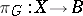such that the groupacts freely and perfectly on the space. The significance of principal fibre bundles lies in the fact that they make it possible to construct associated fibre bundles with fibreif a representation ofin the group of homeomorphismsis given. Differentiable principal fibre bundles with Lie groups play an important role in the theory of connections and holonomy groups. For instance, letbe a topological group withas a closed subgroup and let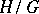be the homogeneous space of left cosets ofwith respect to; the fibre bundle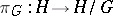will then be principal. Further, let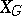be a Milnor construction, i.e. the join of an infinite number of copies of, each point of which has the form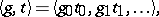where,, and where only finitely many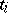are non-zero. The action ofondefined by the formula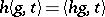is free, and the fibre bundle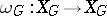is a numerable principal fibre bundle.

Each fibre of a principal fibre bundle is homeomorphic to.

A morphism of principal fibre bundles is a morphism of the fibre bundlesfor which the mapping of the fibres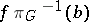induces a homomorphism of groups: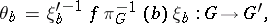where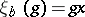,. In particular, a morphism is called equivariant if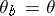is independent of, so that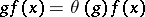for any,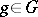. Ifand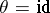, an equivariant morphism is called a-morphism. Any-morphism (i.e. a-morphism over) is called a-isomorphism.

For any mapping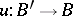and principal fibre bundlethe induced fibre bundle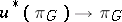is principal with the same group; moreover, the mapping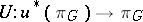is a-morphism which unambiguously determines the action ofon the space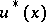. For instance, if the principal fibre bundle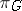is trivial, it is isomorphic to the principal fibre bundle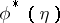, whereis the-bundle over a single point andis the constant mapping. The converse is also true, and for this reason principal fibre bundles with a section are trivial. For each numerable principal fibre bundlethere exists a mapping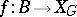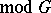such thatis-isomorphic to, and for the principal fibre bundles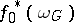and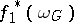to be isomorphic, it is necessary and sufficient that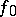and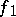be homotopic (cf. Homotopy). This is the principal theorem on the homotopy classification of principal fibre bundles, which expresses the universality of the principal fibre bundle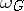(obtained by Milnor's construction), with respect to the classifying mapping.

How to Cite This Entry:
Principal fibre bundle. Encyclopedia of Mathematics. URL: http://encyclopediaofmath.org/index.php?title=Principal_fibre_bundle&oldid=16858
This article was adapted from an original article by A.F. Shchekut'ev (originator), which appeared in Encyclopedia of Mathematics - ISBN 1402006098. See original article